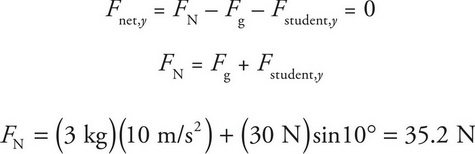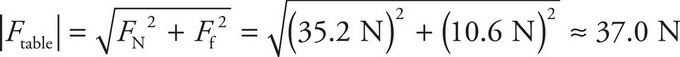# AP Physics 1 Question 382: Answer and Explanation

### Test Information

Question: 382

10. A student is pushing a 3-kg book across a table with a constant force of 30.0 N directed 10° below the horizontal. The coefficient of kinetic friction between the book and the table is 0.3. What is the magnitude of the force that the table exerts on the book during this motion?

• A. 30.7 N
• B. 37.0 N
• C. 38.2 N
• D. 45.0 N

Explanation:

B

There are four forces acting on the book: the push from the student, gravity, normal force, and friction. Of these four forces, normal force and friction are forces exerted by the table on the book. The normal force is calculated from knowing that the net force in the y-direction is zero:The force of friction is calculated using the normal force:

Ff = μFN = (0.3)(35.2 N) = 10.6 N

The force on the book from the table will then be the vector sum of the normal and friction forces. Since the normal force is in the y-direction, and the friction force is in the x-direction, the magnitude of their sum is found using